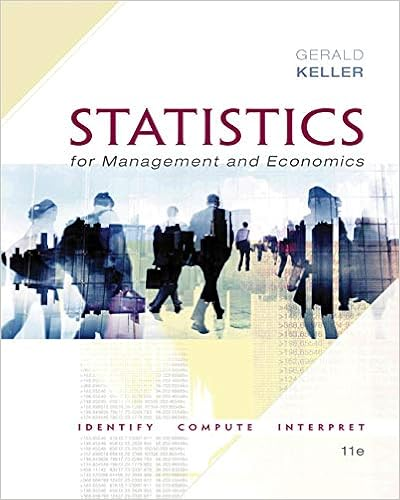# 131 data array and matrix notation in excel data are

• Notes
• 19
• 100% (2) 2 out of 2 people found this document helpful

This preview shows page 3 - 6 out of 19 pages.

##### We have textbook solutions for you!
The document you are viewing contains questions related to this textbook.The document you are viewing contains questions related to this textbook.
Chapter 22 / Exercise 22.8
Statistics for Management and Economics + XLSTAT Bind-in
KellerExpert Verified
1.3.1 Data Array and Matrix Notation In Excel, data are organized as tables or arrays. We express them with matrix notion. A m -by- n matrix A R m × n is an array of elements a ij , i = 1 , . . . , m , j = 1 , . . . , n , in the form of A = [ a ij ] = a 11 a 12 . . . a 1 n a 21 a 22 . . . a 2 n . . . a m 1 a m 2 . . . a mn . 3
##### We have textbook solutions for you!
The document you are viewing contains questions related to this textbook.The document you are viewing contains questions related to this textbook.
Chapter 22 / Exercise 22.8
Statistics for Management and Economics + XLSTAT Bind-in
KellerExpert Verified
All scalars and vectors are special cases of matrices. For example, a row vector c = ( c 1 , c 2 , . . . , c n ) R 1 × n is a 1-by- n matrix; a column vector b = b 1 b 2 . . . b m is a m -by-1 matrix. Let row vector b = ( b 1 , . . . , b n ) be the transpose of column vector b . Let x, b R n . The inner product of vectors c and x is c · x = n i =1 c i x i . The notation Ax b stands for Ax = a 11 a 12 . . . a 1 n a 21 a 22 . . . a 2 n . . . a m 1 a m 2 . . . a mn x 1 x 2 . . . x n = LHS z }| { n j =1 a 1 j x j n j =1 a 2 j x j . . . n j =1 a nj x j RHS z }| { b 1 b 2 . . . b n That is, Ax is a n -by-1 vector, with i -th element being the inner product between the i -th row vector of matrix A and the column vector x . Excel: the inner product c · x =SUMPRODUCT(c,x) . For matrix A , we name the relevant cells as A . To retrieve the element a ij in the i th row and j th column, we use =INDEX(A,i,j) . Also, Ax = MMULT(A,x) (array function, use CTRL+SHIFT+ENTER). 1.3.2 Random Variables, PDF, and CDF A random variable X describes a population , i.e., all possible outcomes of an experiment with probability distribution function (pdf, or pmf) f ( x ) 0 . There are two types of random variables, discrete and continuous. For example, discrete uniform (thraw a die), Bernouli (0-1), Binomial ( n, p ) , and multinomial, Poisson are of discrete type. Continuous uniform, normal N ( μ, σ 2 ) , and exponential random variables are of continuous type. If X is discrete with outcomes { x 1 , . . . , x n } such that f ( x i ) > 0 , then n i =1 f ( x i ) = 1 . If X is continuous with f ( x ) > 0 on interval [ a, b ] , then b a f ( x ) d x = 1 . For a given pdf f ( x ) , we can generate its accumulative distribution function (PDF) F ( x ) : F ( x i ) = i j =1 f ( x j ) = f ( x 1 ) + f ( x 2 ) + · · · + f ( x i ) , i = 1 , 2 , . . . , n, if X is discrete F ( x ) = x a f ( t ) d t = the area under the pdf curve from a up to x, if X is continuous . Excel: We express pdf and cdf by two columns of data: the first column lists the value of possible outcomes x ; the second column gives corresponding f ( x ) (or F ( x ) ). 1.3.3 Generate Random Variables To generate a random variable X from CDF F , we first create a uniform random seed U ∼ U [0 , 1] between 0 and 1; in Excel U = rand () . Then X = F 1 ( U ) . 4
To see why, let G be the CDF of random variable X = F 1 ( U ) . We need to show that G equals F ; i.e., the random variable indeed behave as the function F dictates. Indeed, this is the case: G ( x ) = P { X x } = P { F 1 ( U ) x } by definition X = F 1 ( U ) = P { F ( F 1 ( U )) F ( x ) } since F is increasing = P { U F ( x ) } since F ( F 1 ( U ) ) = U = F ( x ) . since U ∼ U [0 , 1] with CDF F U ( u ) = P ( U u ) = u .
•••